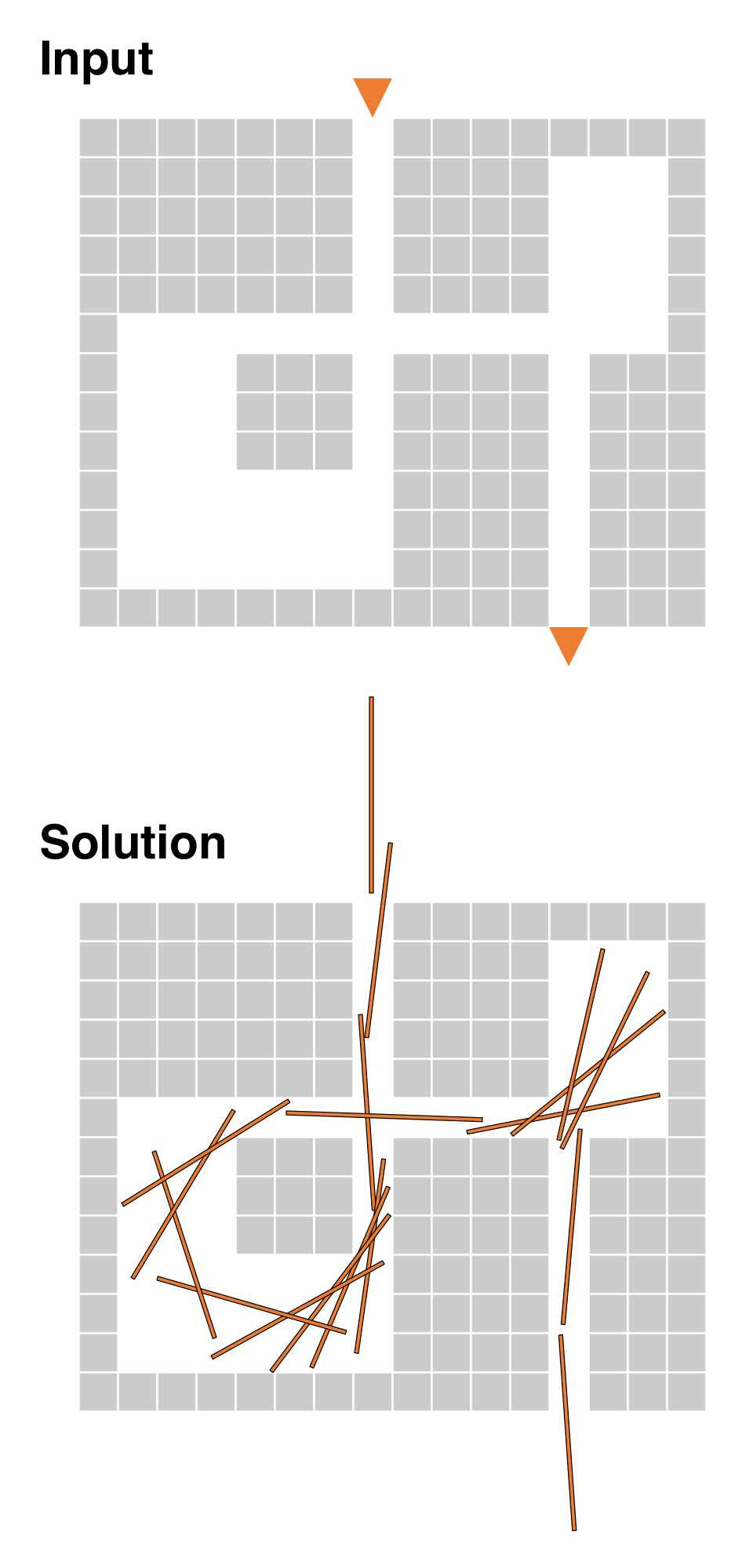CSES - HIIT Open 2017 - Labyrinth
• Time limit: 10.00 s
• Memory limit: 512 MB
You need to transport a stick through a 2-dimensional maze. The length of the stick is 5 meters and the width is 1 centimeter. The maze consists of blocks of size 1×1 meters. You cannot bend the stick, break it, or lift it. You can only slide it along the floor of the maze.Input

The first input line contains an integer $t$: the number of test cases. Then there are $t$ test cases, described as follows:

First there is a line with two numbers, $n$ and $m$, which indicates the size of the maze: $n$ blocks tall and $m$ blocks wide.

Then there is a line with two numbers, $a$ and $b$, indicating the position of the entrance and exit, with $2 \le a \le m-1$, and $2 \le b \le m-1$. The entrance is in the top row, in column $a$, and the exit is in the bottom row, in column $b$.

Finally, there are $n$ lines, with $m$ characters on each row. The characters are either 0 or 1, indicating a block of empty space or a block of solid wall, respectively.

All blocks on the perimeter of the maze are walls, with the exception of the entrance and exit, which are empty.

Output

Output $t$ lines, one per test case. For each test case, output YES if there is a way to navigate the stick through the maze from the entrance to the exit, and NO otherwise.

Limits
• $1 \le t \le 50$
• $3 \le n \le 30$
• $1 \le m \le 30$
Example

Here we have four test cases, the first one is illustrated above.

Input:
4 13 16 8 13 1111111011111111 1111111011110001 1111111011110001 1111111011110001 1111111011110001 1000000000000001 1000111011110111 1000111011110111 1000111011110111 1000000011110111 1000000011110111 1000000011110111 1111111111110111 13 16 8 13 1111111011111111 1111111011110001 1111111011110001 1111111011110001 1111111011110001 1000000000000001 1011111011110111 1011111011110111 1011111011110111 1011111011110111 1011111011110111 1000000011110111 1111111111110111 13 16 8 13 1111111011111111 1111111011111111 1111111011110001 1111111011110001 1111111011110001 1000000000000001 1000111011110111 1000111011110111 1000111011110111 1000000011110111 1000000011110111 1000000011110111 1111111111110111 1 3 2 2 101

Output:
YES NO NO YES